Скачать презентацию Dynamics of Rotatory Motion • Angular Momentum

dynamics_-_rotary_motion.ppt

• Размер: 82.5 Кб
• Количество слайдов: 8

## Описание презентации Dynamics of Rotatory Motion • Angular Momentum по слайдам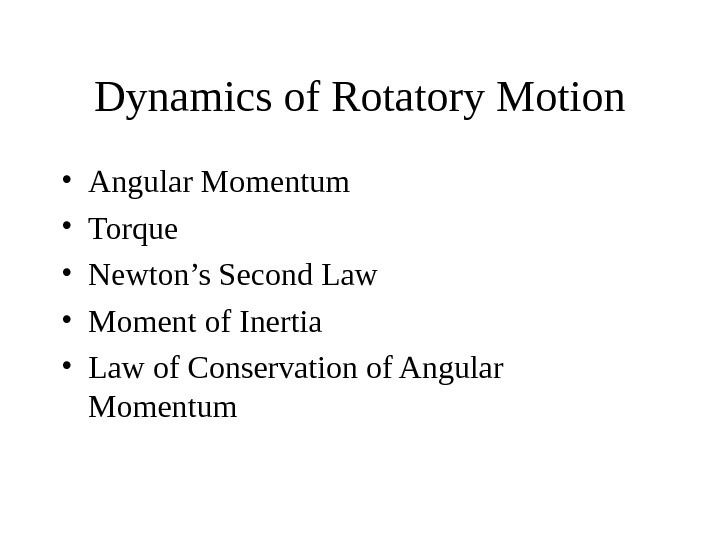Dynamics of Rotatory Motion • Angular Momentum • Torque • Newton’s Second Law • Moment of Inertia • Law of Conservation of Angular Momentum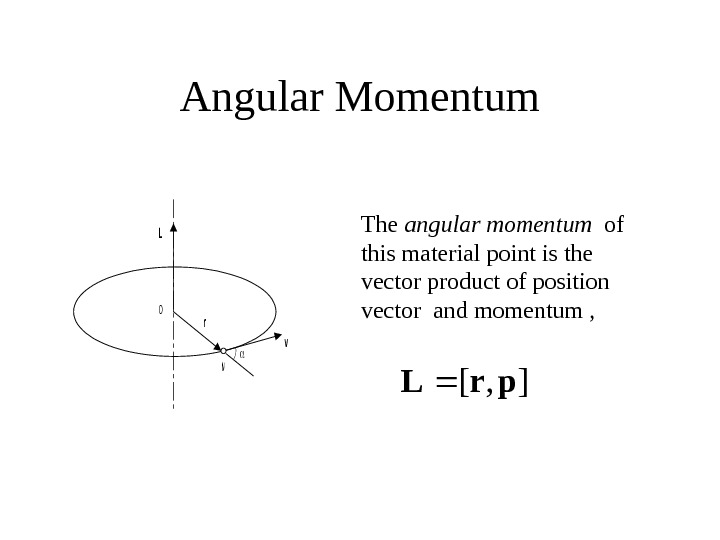Angular Momentum. O v r L M The angular momentum of this material point is the vector product of position vector and momentum , ], [pr. L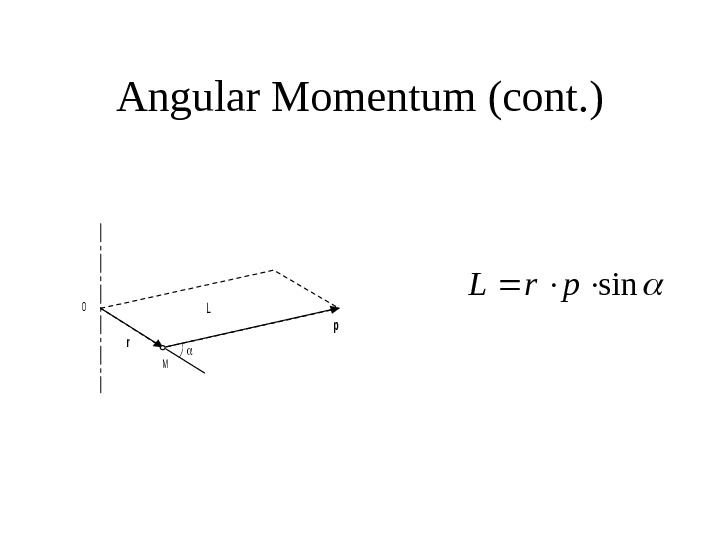Angular Momentum (cont. )O p r M L sinpr. L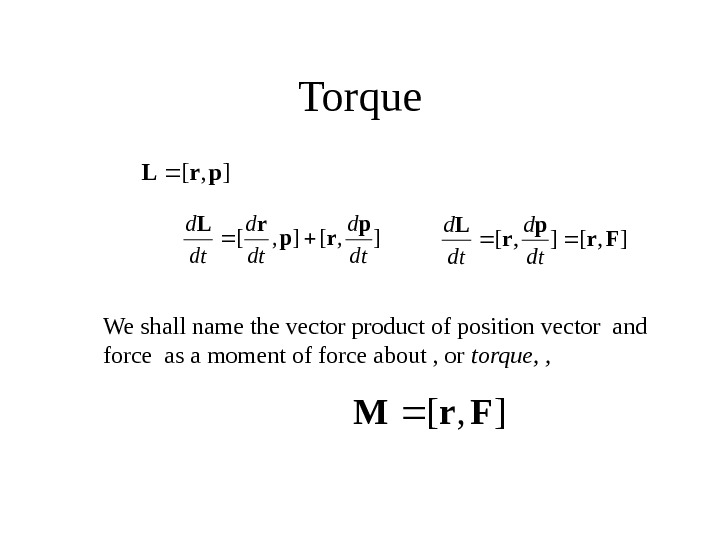Torque], [pr. L ], [ dt dp rp r. L ], [Fr p r L dt d We shall name the vector product of position vector and force as a moment of force about , or torque, , ], [Fr. M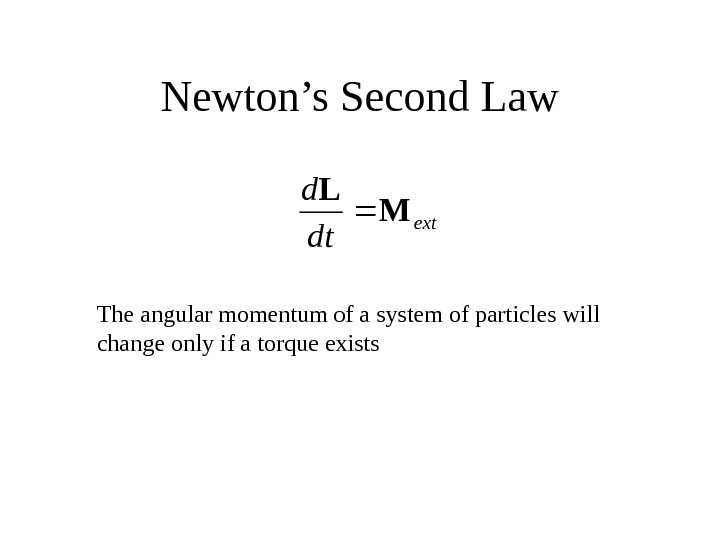Newton’s Second Lawext dt d M L The angular momentum of a system of particles will change only if a torque existsMoment of Inertia z d m v r z. Idmr 2 ωLI The angular momentum of an object is equal to the product of the moment of inertia of this object and its angular velocity dmrdmvr. L z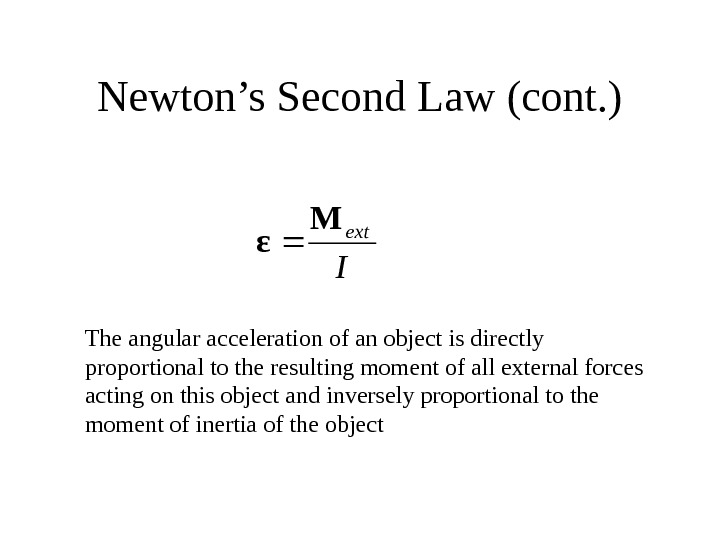Newton’s Second Law (cont. )I ext. M ε The angular acceleration of an object is directly proportional to the resulting moment of all external forces acting on this object and inversely proportional to the moment of inertia of the object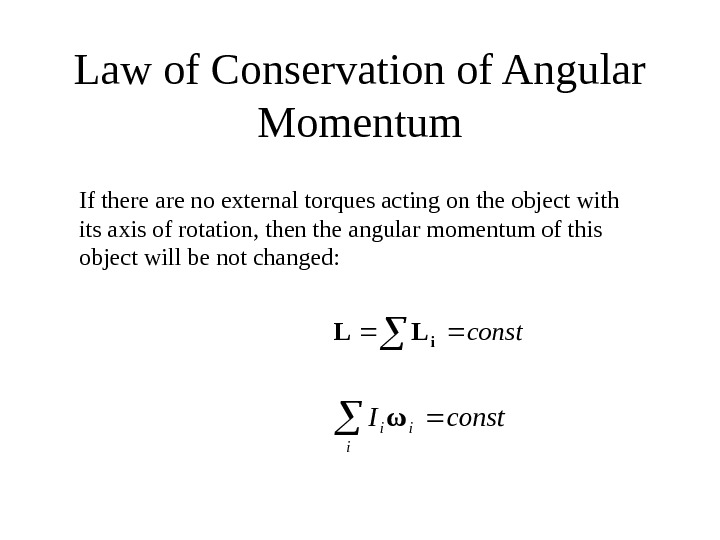Law of Conservation of Angular Momentumconsti. LL i iiconst. Iω If there are no external torques acting on the object with its axis of rotation, then the angular momentum of this object will be not changed: